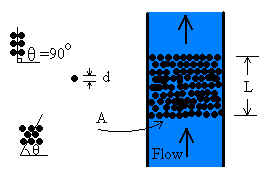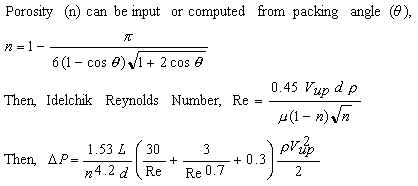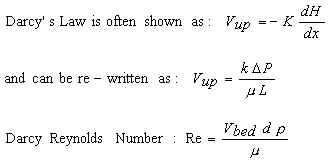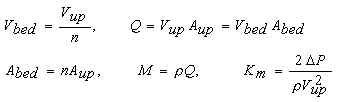Packed Bed Calculator, Porous Media, Permeameter

Bed contains spherical particles. Select Idelchik method or Darcy's Law. Solve for flow, pressure loss, or bed length. Any liquid or gas

Register to enable "Calculate" button.

 Make Selections: Compute M, Q, Vbed Compute P (M known) Compute P (Q known) Compute P (Vbed known) Compute L (M known) Compute L (Q known) Compute L (Vbed known) Idelchik method Darcy Law method Mercury, 20C (68F) Water, 20C (68F) Water, 4C (39F) Seawater, 16C (60F) SAE 30 oil, 16C (60F) Gasoline, 16C (60F) Air, 20C (68F), 1 atm Carbon Dioxide, 20C (68F), 1 atm Nitrogen, 20C (68F), 1 atm Helium, 20C (68F), 1 atm Enter density, viscosity Enter porosity Enter packing angle © 2015 LMNO Engineering, Research, and Software, Ltd. www.LMNOeng.com Pressure Loss, ΔP: m fluid Pascal mbar mm H2O ft fluid psi inch H2O inch Hg Bed Length, L: cm m inch ft Upstream Area, Aup: cm2 m2 inch2 ft2 Mass Flow Rate, M: kg/s kg/hr kg/day kg/yr kg/m2-s kg/m2-hr lb/s lb/hr lb/day lb/yr lb/ft2-s lb/ft2-hr Volumetric Flow, Q: m3/s m3/min ft3/s ft3/min gal/min (US) gal/hr (US) gal/day (US) barrels/day Upstream Velocity, Vup: m/s ft/s Bed Velocity, Vbed: m/s Fluid Density, ρ: g/cm3 kg/m3 N/m3 slug/ft3 lb/ft3 Fluid Viscosity, μ: centipoise, cP poise, dyne-s/cm2 N-s/m2, kg/m-s lb(f)-s/ft2, slug/ft-s centistoke, cSt stoke, cm2/s m2/s ft2/s Grain Diameter, d: mm cm m mil (0.001 inch) inch Bed Porosity, n: Packing Angle, θ: radians degrees Bed Permeability, k: cm2 m2 ft2 darcy Minor Loss Coeff, Km: Idelchik Reynolds Number: Darcy Reynolds Number:Units: barrel=42 U.S. gallons, cm=centimeter, cP=centipoise, cSt=centistoke, darcy=permeability unit (1.01325x1012 darcy=1 m2), inch H2O=inch water at 60F, inch Hg=inch mercury at 60F, ft=foot, ft fluid=ft of whatever fluid is flowing through the bed, g=gram, gal=U.S. gallon, hr=hour, kg=kilogram, kg/m2-s=kg/s of flow through 1 m2 of upstream area, lb=pound, lb/ft2-s=lb/s of flow through 1 ft2 of upstream area, lb(f)=pound (force), m=meter, m fluid=meters of whatever fluid is flowing through the bed, mbar=millibar, mm=millimeter, mm H2O=mm water at 4C, min=minute, N=Newton, psi=lb(f)/in2, s=second

Introduction
Chemical engineers know this as a packed or porous bed. Groundwater hydrologists call this a permeameter or flow through porous media. We provide two methods - Idelchik and Darcy's law - since hydrogeologists and civil engineers are usually more familiar with Darcy's law while chemical and mechanical engineers may be more familiar with the Idelchik approach. The Idelchik method is valid for laminar or turbulent flow through the bed while Darcy's law is valid only for laminar flow (Darcy Reynolds Number<10). Another difference is that only Darcy's law requires entering the permeability. Permeability is used rather than hydraulic conductivity since permeability is a soil property independent of fluid while hydraulic conductivity is defined for water only. If the Idelchik method is selected, permeability will be back-calculated (based on Darcy's law) in case you want to compare a bed material with a soil type. We also compute a minor loss coefficient (Km) for flow through the bed in case the bed is part of a longer pipeline that you are modeling. Any liquid or gas can be used with either method so long as the fluid's density and viscosity are known. Several fluids have properties built into the program.

Equations
Idelchik Method (Fried and Idelchik, 1989):
Valid for any porosity and Reynolds number. However, if porosity is computed from packing angle, the packing angle equation is only valid for packing angles between 60o and 90o (resulting in computed porosity between 0.2595 and 0.4764).Darcy's Law Method (e.g. Freeze and Cherry, 1979; Bear, 1979):
Valid for Darcy Reynolds Number < 10 and any porosity. Porosity can be computed from packing angle from Idelchik's equation above for packing angles between 60o and 90o.For Both Methods:For our calculation, all equations were solved analytically for the desired variables, except when computing velocity and flowrate using the Idelchik method. This required a numerical solution based on a cubic solver such as presented in Rao (1985).

Variables  (Units: F=Force, L=Length, M=Mass, T=Time)
Aup=Upstream area (column cross-sectional area) [L2].
Abed=Bed area (column cross-sectional area minus area of grains) [L2].
d=Grain diameter [L].
k=Permeability of particles [L2].
K=Hydraulic conductivity [L/T]. Not used in calculation but shown in equations above. Instead of K, k is used in calculations since it is independent of fluid type.
Km=Minor loss coefficient of bed [unit-less].
L=Bed length [L]. Also known as permeameter length.
M=Mass flow rate [M/T].
n=Porosity of bed. Enter as decimal. For example, enter 35% as 0.35.
Q=Volumetric flow rate [L3/T].
Re(Idelchik)=Reynolds number defined by Idelchik method [unit-less]. Based on upstream velocity.
Re(Darcy)=Reynolds number for Darcy method [unit-less]. Based on bed velocity.
Vup=Upstream velocity [L/T]. Also known as Darcy velocity. Upstream of porous bed.
Vbed=Bed velocity [L/T]. Velocity of fluid flowing through the bed. Also known as pore velocity.
ρ=Fluid density [M/L3].
μ=Fluid dynamic viscosity [F-T/L2] or [M/L-T].
ΔP=Pressure loss [F/L2]. Upstream Pressure - Downstream Pressure.
θ=Packing angle [radians or degrees]. Angle between successive rows of particles.

Soil Properties
The following table of soil properties was compiled from Freeze and Cherry (1979), Bear (1979), Hillel (1982), and Sanders (1998).

Table of Soil Properties

 Soil Type Permeability, k (m2) Porosity, n Grain Size, d (mm) Clayey 10-18 - 10-15 0.4 - 0.7 < 0.002 Silty 10-16 - 10-12 0.35 - 0.5 0.002 - 0.05 Sandy 10-14 - 10-10 0.25 - 0.5 0.05 - 2 Gravelly 10-10 - 10-7 0.25 - 0.4 > 2

Error Messages given by calculation
Input checks.  If one of these messages appear, the calculation will not proceed.
Lower limits on variables:
"A must be > 0", "Density must be > 0", "Viscosity must be > 0", "Grain dia must be > 0", "L must be > 0", "k must be > 0", "Pressure loss must be > 0", "M must be > 0", "Q must be > 0", "Vbed must be > 0".

Upper limits on variables:
"A must be < 1e50 m2", "Density must be < 1e6 kg/m3", "Viscosity must be < 1e9 m2/s", "d must be < 1000 m", "L must be < 1e10 m", "k must be < 100 m2", "P loss must be < 1e50 Pa", "M must be < 1e50 kg/s", "Q must be < 1e50 m3/s", "Vbed must be < 1e50 m/s".

Other limits on variables:
"Porosity out of range".  Porosity must be between 0.00001 and 0.99999.
"Angle out of range".  If packing angle is entered, it must be between 60o and 90o.
"L must be ≥ d".  Bed length cannot be less than grain size.

Run-time errors.  The following messages may be generated during execution:
"Infeasible input."  May occur when using Idelchik method and solving for flowrate using input values that approach machine precision (e.g. Pressure loss of 10-200 Pa) and are physically unrealistic.
"L will be < d."  May appear if bed length is being computed, and the length will be less than the grain diameter.
"Darcy Re >10."  Will appear only if the Darcy method was selected, and the Darcy Reynolds number is > 10.  Darcy's law is only valid for laminar flow which is defined as Re(Darcy)<10.  This is only a warning message; results will be computed and shown.

References
Bear, J. 1979. Hydraulics of Groundwater. McGraw-Hill Pub Co.

Freeze, R. A. and J. A. Cherry. 1979. Groundwater. Prentice Hall, Inc.

Fried, E. and I. E. Idelchik. 1989. Flow Resistance: A Design Guide for Engineers. Hemisphere Pub. Corp.

Hillel, D. 1982. Introduction to Soil Physics. Academic Press, Inc.

Rao, S. S. 1985. Optimization Theory and Applications. Wiley Eastern Limited. 2ed.

Sanders, L. L. 1998. A Manual of Field Hydrogeology. Prentice Hall, Inc.

LMNO Engineering, Research, and Software, Ltd.
7860 Angel Ridge Rd.   Athens, Ohio 45701  USA   Phone: (740) 592-1890
LMNO@LMNOeng.com    http://www.LMNOeng.com

To:

Related Calculations: